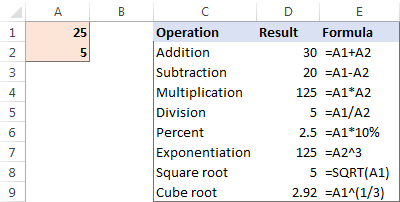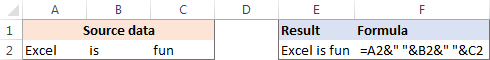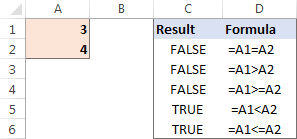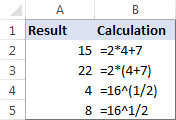# How to do calculations in Excel

The tutorial shows how to do arithmetic calculations in Excel and change the order of operations in your formulas.

When it comes to calculations, there is almost noting that Microsoft Excel cannot do, from totaling a column of numbers to solving complex linear programming problems. For this, Excel provides a few hundred predefined formulas, called Excel functions. In addition, you can use Excel as a calculator to do math - add, divide, multiply, and subtract numbers as well as raise to power and find roots.

## How to do calculations in Excel

Making calculations in Excel is easy. Here's how:

• Type the equal symbol (=) in a cell. This tells Excel that you are entering a formula, not just numbers.
• Type the equation you want to calculate. For example, to add up 5 and 7, you type =5+7
• Press the Enter key to complete your calculation. Done!

Instead of entering numbers directly in your calculation formula, you can put them in separate cells, and then reference those cells in your formula, e.g. =A1+A2+A3

The following table shows how to perform basic arithmetic calculations in Excel.

 Operation Operator Example Description Addition + (plus sign) =A1+A2 Adds up the numbers in cells A1 and A2. Subtraction - (minus sign) =A1-A2 Subtracts the number in A2 from the number in A1. Multiplication * (asterisk) =A1*A2 Multiplies the numbers in A1 and A2. Division / (forward slash) =A1/A2 Divides the number in A1 by the number in A2. Percent % (percent) =A1*10% Finds 10% of the number in A1. Raising to power (Exponentiation) ^ (caret) =A2^3 Raises the number in A2 to the power of 3. Square root SQRT function =SQRT(A1) Finds the square root of the number in A1. Nth root ^(1/n) (Where n is the root to find) =A1^(1/3) Finds the cube root of the number in A1.

The results of the above Excel calculation formulas may look something similar to this:Apart from that, you can combine values from two or more cells in a single cell by using the concatenation operator (&) like this:

`=A2&" "&B2&" "&C2`

A space character (" ") is concatenated in between cells to separate the words:You can also compare cells by using logical operators such as "greater than" (>), "less than" (<), "greater than or equal to" (>=), and "less than or equal to" (<=). The result of comparison are logical values of TRUE and FALSE:## The order in which Excel calculations are performed

When you do two or more calculations in a single formula, Microsoft Excel calculates the formula from left to right, according to the order of operations shown in this table:

 Precedence Operation 1 Negation, i.e. reversing the number sign, as in -5, or -A1 2 Percent (%) 3 Exponentiation, i.e. raising to power (^) 4 Multiplication (*) and division (/), whichever comes first 5 Addition (+) and subtraction (-), whichever comes first 6 Concatenation (&) 7 Comparison (>, <, >=, <=, =)

Since the order of calculations affects the final result, you need to know how to change it.

## How to change the order of calculations in Excel

Like you do in math, you can change the order of Excel calculations by enclosing the part to be calculated first in parentheses.

For example, the calculation `=2*4+7` tells Excel to multiply 2 by 4, and then add 7 to the product. The result of this calculation is 15. By enclosing the addition operation in parentheses `=2*(4+7)`, you instruct Excel to add up 4 and 7 first, and then multiply the sum by 2. And the result of this calculation is 22.

Another example is finding a root in Excel. To get the square root of, say, 16, you can use either this formula:

`=SQRT(16)`

or an exponent of 1/2:

`=16^(1/2)`

Technically, the above equation tells Excel to raise 16 to the power of 1/2. But why do we enclose 1/2 in parentheses? Because if we don't, Excel will raise 16 to the power of 1 first (an exponent operation is performed before division), and then divide the result by 2. Since any number raised to the power of 1 is the number itself, we would end up dividing 16 by 2. In contrast, by enclosing 1/2 in parentheses you tell Excel to divide 1 by 2 first, and then raise 16 to the power of 0.5.

As you can see in the screenshot below, the same calculation with and without parentheses produces different results:This is how you make calculations in Excel. I thank you for reading and hope to see you on our blog next week!